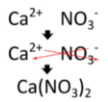# 3.9: Names and Formulas of Ternary Ionic Compounds

$$\newcommand{\vecs}{\overset { \rightharpoonup} {\mathbf{#1}} }$$ $$\newcommand{\vecd}{\overset{-\!-\!\rightharpoonup}{\vphantom{a}\smash {#1}}}$$$$\newcommand{\id}{\mathrm{id}}$$ $$\newcommand{\Span}{\mathrm{span}}$$ $$\newcommand{\kernel}{\mathrm{null}\,}$$ $$\newcommand{\range}{\mathrm{range}\,}$$ $$\newcommand{\RealPart}{\mathrm{Re}}$$ $$\newcommand{\ImaginaryPart}{\mathrm{Im}}$$ $$\newcommand{\Argument}{\mathrm{Arg}}$$ $$\newcommand{\norm}{\| #1 \|}$$ $$\newcommand{\inner}{\langle #1, #2 \rangle}$$ $$\newcommand{\Span}{\mathrm{span}}$$ $$\newcommand{\id}{\mathrm{id}}$$ $$\newcommand{\Span}{\mathrm{span}}$$ $$\newcommand{\kernel}{\mathrm{null}\,}$$ $$\newcommand{\range}{\mathrm{range}\,}$$ $$\newcommand{\RealPart}{\mathrm{Re}}$$ $$\newcommand{\ImaginaryPart}{\mathrm{Im}}$$ $$\newcommand{\Argument}{\mathrm{Arg}}$$ $$\newcommand{\norm}{\| #1 \|}$$ $$\newcommand{\inner}{\langle #1, #2 \rangle}$$ $$\newcommand{\Span}{\mathrm{span}}$$$$\newcommand{\AA}{\unicode[.8,0]{x212B}}$$Figure $$\PageIndex{1}$$ (Credit: Jon Sullivan; Source: Commons Wikimedia, Pepperoni Pizza(opens in new window) [commons.wikimedia.org]; License: Public Domain)

### How many varieties of pizza can you think of?

Many people enjoy eating pizza. When someone orders a pizza (either by phone, online, or in a restaurant), they don't just order "pizza". Even the simplest "binary" pizza will have a topping and cheese—maybe pepperoni, maybe something else. However, many pizzas quickly become more complex. One person may want pepperoni and sausage, another may wish to order Canadian bacon and pineapple; then you have the folks that can't decide, so they order half-this and half-that. The combinations may be more complex, but the same basic ideas about pizza are valid.

## Ternary Ionic Compounds

Not all ionic compounds are composed of only monatomic ions. A ternary ionic compound is an ionic compound composed of three elements. In a typical ternary ionic compound, there is still one type of cation and one type of anion involved. The cation, the anion, or both, are polyatomic ions.

## Naming Ternary Ionic Compounds

The process of naming ternary ionic compounds is the same as naming binary ionic compounds. The cation is named first, followed by the anion. Some examples are shown in the table below:

Formula Name
Table $$\PageIndex{1}$$: Examples of Ternary Ionic Compounds
$$\ce{NaNO_3}$$ sodium nitrate
$$\ce{NH_4Cl}$$ ammonium chloride
$$\ce{Fe(OH)_3}$$ iron (III) hydroxide

When more than one polyatomic ion is present in a compound, the formula of the ion is placed in parentheses with a subscript outside of the parentheses that indicates how many of those ions are in the compound. In the last example above, there is one $$\ce{Fe^{3+}}$$ cation and three $$\ce{OH^-}$$ anions.

## Writing Formulas for Ternary Ionic Compounds

Writing a formula for a ternary ionic compound also involves the same steps as for a binary ionic compound. Write the symbol and charge of the cation followed by the symbol and charge of the anion. Use the crisscross method to ensure that the final formula is neutral. Calcium nitrate is composed of a calcium cation and a nitrate anion.Figure $$\PageIndex{2}$$ (Credit: Joy Sheng; Source: CK-12 Foundation; License: CC BY-NC 3.0(opens in new window))

The charge is balanced by the presence of two nitrate ions and one calcium ion. Parentheses are used around the nitrate ion because more than one of the polyatomic ion is needed. If only one polyatomic ion is in a formula, parentheses are not used. As an example, the formula for calcium carbonate is $$\ce{CaCO_3}$$. The carbonate ion carries a $$2-$$ charge and so exactly balances the $$2+$$ charge of the calcium ion.

There are two polyatomic ions that produce unusual formulas. The $$\ce{Hg_2^{2+}}$$ ion is called either the dimercury ion or, preferably, the mercury (I) ion. When bonded with an anion with a $$1-$$ charge, such as chloride, the formula is $$\ce{Hg_2Cl_2}$$. Because the cation consists of two $$\ce{Hg}$$ atoms bonded together, this formula is not reduced to $$\ce{HgCl}$$. Likewise, the peroxide ion, $$\ce{O_2^{2-}}$$, is also a unit that must stay together in its formulas. For example, the formula for potassium peroxide is $$\ce{K_2O_2}$$.

## Summary

• Ternary compounds are composed of three or more elements.
• Ternary compounds are named by stating the cation first, followed by the anion.
• Positive and negative charges must balance.

## Review

1. What is a ternary compound?
2. What is the basic rule for naming ternary compounds?
3. Write the formulas for the following compounds:
1. mercury(II) nitrate
2. ammonium phosphate
3. calcium silicate